13 min read

# Ecospat Niche Overlap Analysis

§sdm §spatial

When comparing ranges (geographic or environmental) between two datasets (e.g., the same species in different times/regions, to assess invasion patterns), we can use the ecospat program to calculate indexes that quantify expansion (area occupied in the invaded range but not the native), stability (area occupied in both native and invaded), and infilling (area occupied only in the native range, not the invaded). Note that depending on the context, ‘native’ and ‘invaded’ may in fact refer to different species being compared, rather than the same species being compared in different contexts.

# Setup

In order to use the most recent updates to ecospat, downloaded from their Github repository:

setwd("~/hacking/ecospat/ecospat")
library(devtools)
load_all()

# Data Preparation

Required preliminary data are ordination scores for the total environment (i.e., the entire range of both species), the local environment (i.e., the ranges of each species of interest), and the PCA scores for each species of interest. The ordination space is defined by the background environment, the species scores are supplemental.

data(ecospat.testNiche)
data(ecospat.testData)

## The coordinates for two different species:
sp1 <- subset(ecospat.testNiche,
Spp == levels(ecospat.testNiche$Spp)) sp2 <- subset(ecospat.testNiche, Spp == levels(ecospat.testNiche$Spp))

## The environmental data for 300 locations:
clim <- ecospat.testData[2:8]

## Add environmental data to species data:
sp1 <- cbind(sp1,
na.exclude(
ecospat.sample.envar(dfsp = sp1, colspxy = 2:3,
colspkept = 1:3,
dfvar = clim,
colvarxy = 1:2,
colvar = "all",
resolution = 25)))

sp2 <- cbind(sp2,
na.exclude(
ecospat.sample.envar(dfsp = sp2, colspxy = 2:3,
colspkept = 1:3,
dfvar = clim,
colvarxy = 1:2,
colvar = "all",
resolution = 25)))

##############################################################
## NB. ecospat.sample.envar samples from a data.frame of  ##
## points, analogously to how extract samples from raster ##
## layer.                                                   ##
##############################################################

## selection of variables to include in the analyses
Xvar <- c("ddeg", "mind", "srad", "slp", "topo")
nvar <- length(Xvar)

pca.cal <- dudi.pca(clim[, Xvar], center = TRUE,
scale = TRUE, scannf = FALSE, nf = 2)

## Species scores:
sp1.scores <- suprow(pca.cal, sp1[, Xvar])$li sp2.scores <- suprow(pca.cal, sp2[, Xvar])$li
scores.clim <- pca.cal$li ## scores.sp1 and scores.sp2 now contain the position of ## these two species in the environmental ordination space. Let’s take a look at the starting data: plot(scores.clim, pch = 16, asp = 1, col = adjustcolor(1, alpha.f = 0.2), cex = 2, xlab = "PC1", ylab = "PC2") points(sp1.scores, pch = 17, col = 2, cex = 2) points(sp2.scores, pch = 18, col = 4, cex = 2)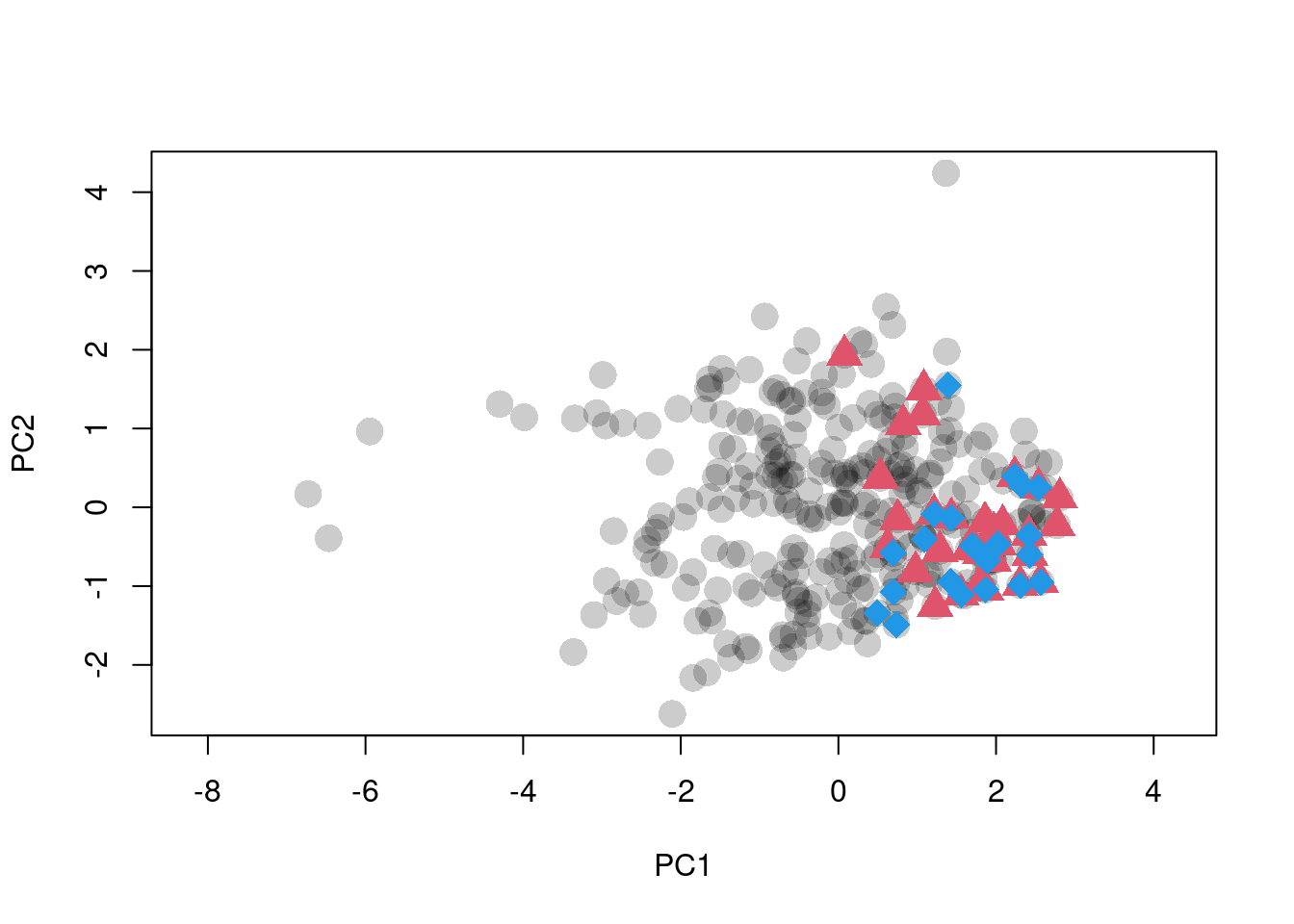Figure 1: Ordination of raw climate data. Black dots are background points. Red triangles and blue diamonds are species occurences. # Kernel Density Estimates Next we create the occurence density grids for the two species we wish to compare. These grids are called gridclim objects in the ecospat documentation (not really objects in the R sense, just lists). We feed the ordination data to ecospat.grid.clim.dyn to get the actual grids: z1 <- ecospat.grid.clim.dyn(scores.clim, scores.clim, sp1.scores, R = 100) z2 <- ecospat.grid.clim.dyn(scores.clim, scores.clim, sp2.scores, R = 100) This does the following: • the extent is set to the limits of the global environment • the kernel density of the local environment is estimated • the kernel density of the species occurence environment is estimated Various transformations and corrections are applied to these estimates. The list that is returned contains: • x, y : the coordinates for each cell in the resulting density plot • z : the occurence density estimate for each cell in the plot, scaled to the total number of occurences in the raw data • z.uncor : z scaled to 0-1. i.e., the density estimate for a cell is a direct reflection of the number of occurences in the vicinity of that cell, regardless of the environmental distribution. • z.cor : z corrected for environmental prevalence i.e., we take the density estimate, and divide by the relative abundance of the environment at that location. Effectively, we need more occurences at common environments to achieve the same density estimate as a smaller number in a rare environment. • Z : the home/local environment density estimate for each cell in the plot, scaled to the total number of rows in the raw environment data (background) • w : the “niche envelope”, which is z.uncor converted to a presence-absence map (all values > 0 become present). • glob, glob1, sp : the coordinates of all observations in the raw global environment, raw local environment, and species occurence data The key outputs are z.uncor and z.cor. We can compare these with a few plots: par(mfrow = c(2, 2), mar = c(0.5, 0.5, 2, 0)) plot(z1$z.uncor, legend = FALSE, axes = FALSE,
main = "z.uncor - Full")
points(z1$sp, pch = 16, col = adjustcolor(1, alpha.f = 0.5)) plot(z1$z.cor, legend = FALSE, main = "z.cor - Full",
axes = FALSE)
points(z1$sp, pch = 16, col = adjustcolor(1, alpha.f = 0.5)) plot(z1$z.uncor, xlim = c(0, 3), ylim = c(-2, 2),
axes = FALSE, legend = FALSE, main = "z.uncor - Zoom")
points(z1$sp, pch = 16, col = adjustcolor(1, alpha.f = 0.5)) plot(z1$z.cor, xlim = c(0, 3), ylim = c(-2, 2), axes = FALSE,
legend = FALSE, main = "z.cor - Zoom")
points(z1$sp, pch = 16, col = adjustcolor(1, alpha.f = 0.5))We can see that z-uncor looks like we expect. The observations are arranged in a circular cloud, which is captured as a circular-shaped kernel density. In contrast, z.cor shows a higher kernel density estimate in the lower right corner, despite using the same observations. The reason is that the observations in the lower right occur in rarer environments, so they are given higher relative weight in the density estimate. Here’s the environmental density: plot(z1$Z, axes = FALSE, legend = FALSE,
main = "Environmental density - Full")
points(z1$sp, pch = 16, col = adjustcolor(1, alpha.f = 0.5))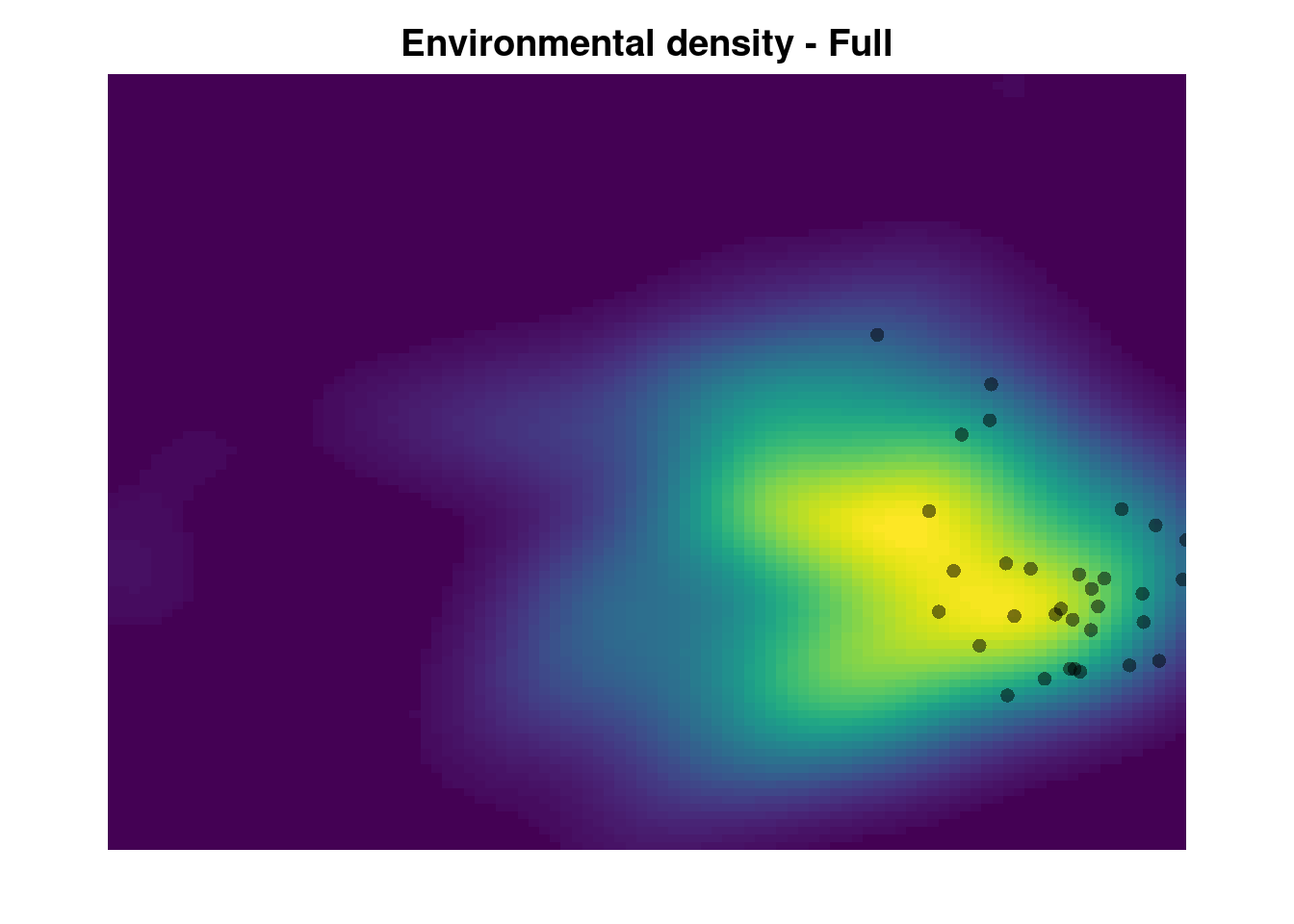The green area shows the most common environments. The observations within this area are given reduced weight relative to the observations in rare environments (the pink areas on the margin). This creates the ‘skew’ in the corrected occurence density kernel. ## Kernel Density Calculation Taking a closer look: The kernel densities are generated from: glob1.dens <- ecospat.kd(x = glob1, ext = ext, method = kernel.method, th = 0) where glob1 is the environmental background fro species 1, ext a vector with min and max values for each PC axis, and kernel.method is set to adehabitat by default, sends the data to adehabitatHR::kernelUD. Let’s extract all the relevant code: R <- 100 # grid size xmin <- apply(scores.clim, 2, min, na.rm = T) xmax <- apply(scores.clim, 2, max, na.rm = T) ext <- c(xmin, xmax, xmin, xmax) ## Shift the raw data to match the extent: xr <- data.frame( cbind( (scores.clim[, 1] - ext) / abs(ext - ext), (scores.clim[, 2] - ext) / abs(ext - ext))) mask <- adehabitatMA::ascgen( sp::SpatialPoints(cbind((0:(R)) / R, (0:(R) / R))), nrcol = R - 2, count = FALSE) x.dens <- adehabitatHR::kernelUD(sp::SpatialPoints(xr[, 1:2]), h = "href", grid = mask, kern = "bivnorm" ) ## Shift the density back to match the original projection: x.dens <- raster::raster( xmn = ext, xmx = ext, ymn = ext, ymx = ext, matrix(x.dens$ud, nrow = R)
)

plot(x.dens)
points(scores.clim)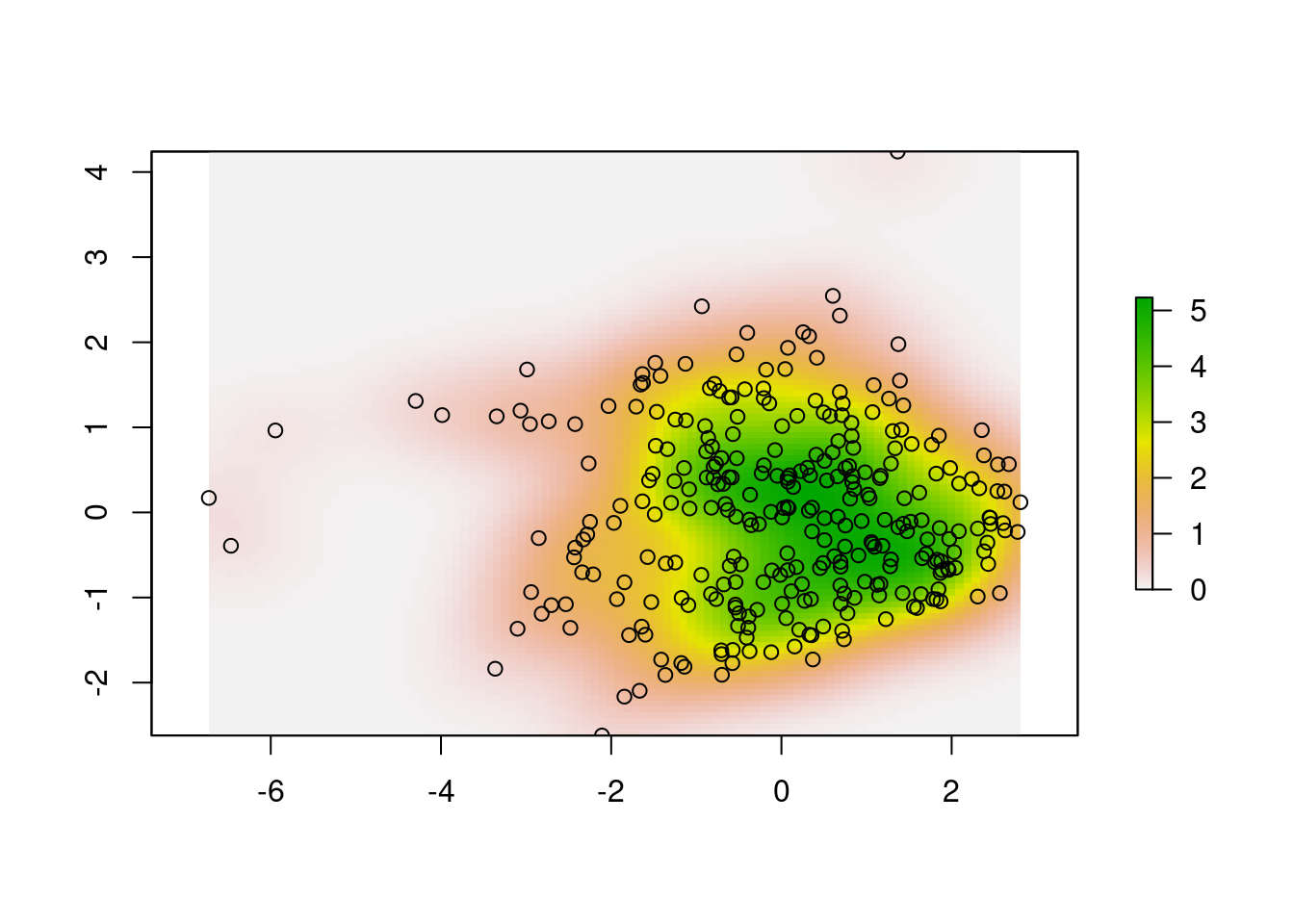We can’t alter the arguments passed to kernelUD, which are set to h = "href" (the smoothing parameter), and kern = "bivnorm" (the kernel function). From , the kernel function isn’t as important as the smoothing parameter. The actual combination chosen depends on the needs of the study. There is an unavoidable tradeoff between simple, general estimates, show the overall pattern of distribution, but at the cost of ‘smoothing away’ data in the tails, vs more accurate models that retain information in the tails, but may over-emphasize the importance of noise in the data.

We could use either the ad hoc smoothing parameter (the default, “href”), or the least-squares cross-validation smoother (“LSCV”). How much difference would it make:

x.dens.ls <- adehabitatHR::kernelUD(sp::SpatialPoints(xr[, 1:2]),
h = "LSCV", grid = mask,
kern = "bivnorm"
)
x.dens.ls <- raster::raster(
xmn = ext, xmx = ext, ymn = ext,
ymx = ext, matrix(x.dens.ls$ud, nrow = R) ) #dev.new(width = 6, height = 8) par(mfrow = c(2, 2), mar = c(2, 0.1, 3, 0)) plot(x.dens, main = "Ad Hoc", legend = FALSE, axes = FALSE) points(scores.clim, pch = 16, cex = 0.5, col = adjustcolor("black", alpha.f = 0.1)) plot(x.dens.ls, main = "LSCV", legend = FALSE, axes = FALSE) points(scores.clim, pch = 16, cex = 0.5, col = adjustcolor("black", alpha.f = 0.1)) plot(x.dens, main = "Ad Hoc", legend = FALSE, axes = FALSE, xlim = c(-1, 2), ylim = c(-1, 1)) plot(x.dens.ls, main = "LSCV", legend = FALSE, axes = FALSE, xlim = c(-1, 2), ylim = c(-1, 1))In the zoomed image, we can see a bit more definition in the LSCV density estimate, but it’s a very slight difference. In this case, there is a lot of data. What happens for the species data, which is sparser? ## Shift the raw data to match the extent: spec1r <- data.frame( cbind( (sp1.scores[, 1] - ext) / abs(ext - ext), (sp1.scores[, 2] - ext) / abs(ext - ext))) sp1.dens <- adehabitatHR::kernelUD(sp::SpatialPoints(spec1r[, 1:2]), h = "href", grid = mask, kern = "bivnorm") sp1.dens <- raster::raster( xmn = ext, xmx = ext, ymn = ext, ymx = ext, matrix(sp1.dens$ud, nrow = R))

sp1.dens.ls <- adehabitatHR::kernelUD(sp::SpatialPoints(spec1r[, 1:2]),
h = "LSCV", grid = mask,
kern = "bivnorm")
sp1.dens.ls <- raster::raster(
xmn = ext, xmx = ext, ymn = ext,
ymx = ext, matrix(sp1.dens.ls$ud, nrow = R)) par(mfrow = c(2, 2), mar = c(2, 0.1, 3, 0)) plot(sp1.dens, main = "Ad Hoc", legend = FALSE, axes = FALSE) points(sp1.scores, pch = 16, cex = 0.5, col = adjustcolor("black", alpha.f = 0.1)) plot(sp1.dens.ls, main = "LSCV", legend = FALSE, axes = FALSE) points(sp1.scores, pch = 16, cex = 0.5, col = adjustcolor("black", alpha.f = 0.1)) plot(sp1.dens, main = "Ad Hoc", legend = FALSE, axes = FALSE, xlim = c(-1, 1), ylim = c(1, 2.5)) points(sp1.scores, pch = 16, cex = 0.5, col = adjustcolor("black", alpha.f = 0.1)) plot(sp1.dens.ls, main = "LSCV", legend = FALSE, axes = FALSE, xlim = c(-1, 1), ylim = c(1, 2.5)) points(sp1.scores, pch = 16, cex = 0.5, col = adjustcolor("black", alpha.f = 0.1))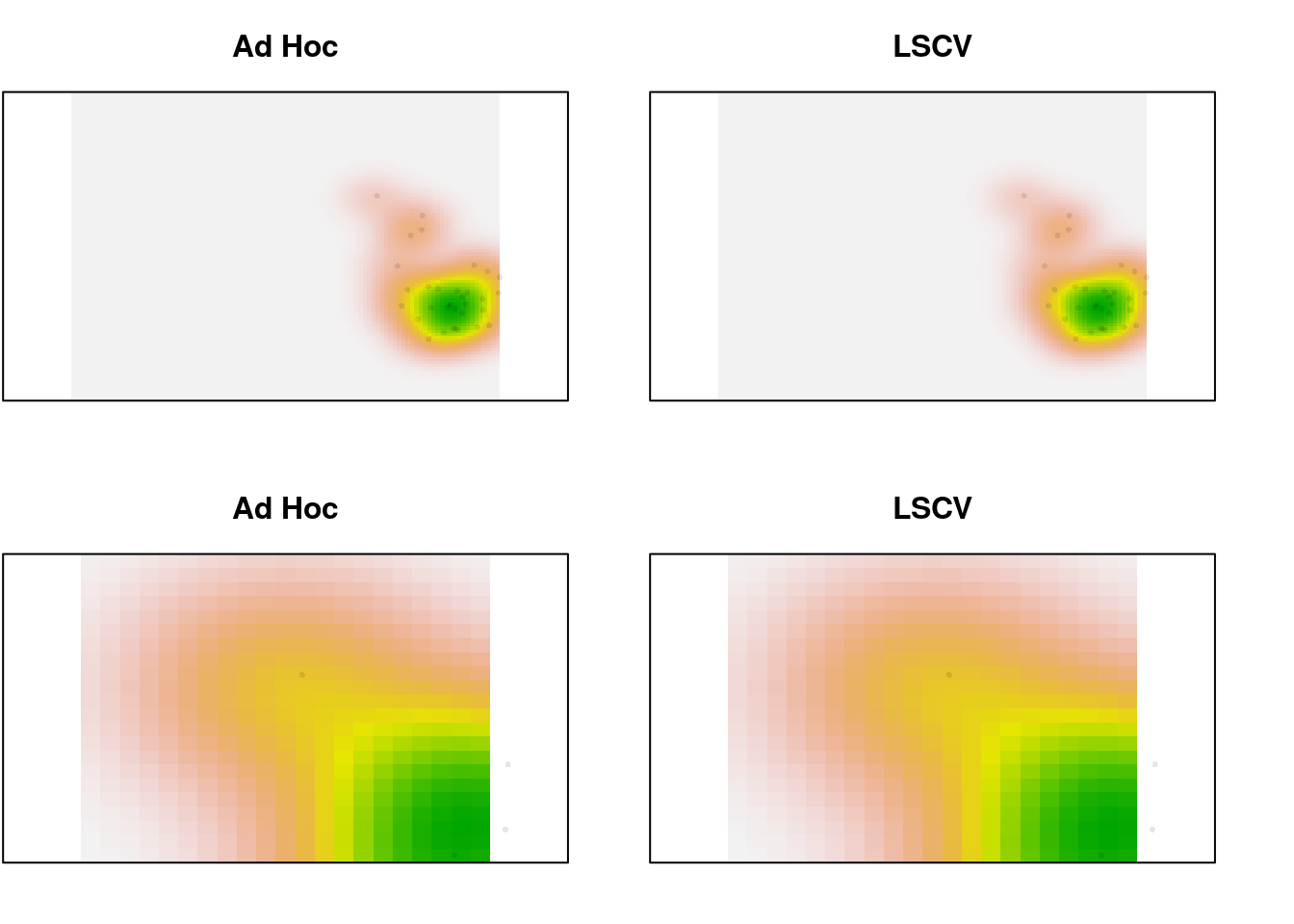Not much difference, even with 32 points. Trim it to just the first 10 and look again: ## Shift the raw data to match the extent: sp1.trim <- sp1.scores[1:7, ] trimr <- data.frame( cbind( (sp1.trim[, 1] - ext) / abs(ext - ext), (sp1.trim[, 2] - ext) / abs(ext - ext))) trim.dens <- adehabitatHR::kernelUD(sp::SpatialPoints(trimr[, 1:2]), h = "href", grid = mask, kern = "bivnorm") trim.dens <- raster::raster( xmn = ext, xmx = ext, ymn = ext, ymx = ext, matrix(trim.dens$ud, nrow = R))

trim.dens.ls <- adehabitatHR::kernelUD(sp::SpatialPoints(trimr[, 1:2]),
h = "LSCV", grid = mask,
kern = "bivnorm")
trim.dens.ls <- raster::raster(
xmn = ext, xmx = ext, ymn = ext,
ymx = ext, matrix(trim.dens.ls$ud, nrow = R)) par(mfrow = c(2, 2), mar = c(2, 0.1, 3, 0)) xlims <- c(0, 5) ylims <- c(-1, 2) plot(trim.dens, main = "Ad Hoc", legend = FALSE, axes = FALSE) points(sp1.trim, pch = 16, cex = 1, col = adjustcolor("black", alpha.f = 0.4)) plot(trim.dens.ls, main = "LSCV", legend = FALSE, axes = FALSE) points(sp1.trim, pch = 16, cex = 1, col = adjustcolor("black", alpha.f = 0.4)) plot(trim.dens, main = "Ad Hoc", legend = FALSE, axes = FALSE, xlim = xlims, ylim = ylims) points(sp1.trim, pch = 16, cex = 1, col = adjustcolor("black", alpha.f = 0.4)) plot(trim.dens.ls, main = "LSCV", legend = FALSE, axes = FALSE, xlim = xlims, ylim = ylims) points(sp1.trim, pch = 16, cex = 1, col = adjustcolor("black", alpha.f = 0.4))Still a pretty subtle difference, even with 32 points. # Niche expansion, stability, and unfilling Once we have our grids, we proceed to calculate the dynamic niche indices. Assume we’re comparing two species, sp1 and sp2, each with their own ‘native’ environment env1 and env2. The global environment globenv is just env1 and env2 combined. In the case of species with the same range (i.e., comparing 2 North American species), env1 = env2 = globenv. We have all the grids available now: w, z.cor, z.uncor, Z, for both species, in the results returned by ecospat.grid.clim.dyn. We can pass this on to ecospat.niche.dyn.index to generate the metrics of interest: ind1 <- ecospat.niche.dyn.index(z1, z2) The first step in this process is categorizing each cell in the ordination as expansion, stability, or unfilling. This is determined using only the presence/absence layers, w: ## Convert to matrices to allow us to do logical operations: w1 <- as.matrix(z1$w)
w2 <- as.matrix(z2$w) stability <- w1 & w2 ## both present expansion <- w2 & ! w1 ## only sp2 present unfilling <- w1 & ! w2 ## only sp1 present ## Combine: expansion = 1, unfilling = 2, stablility = 3) dyn.index <- expansion + (2 * unfilling) + (3 * stability) ## rotate the matrix so the image is oriented properly: dyn.index <- t(apply(dyn.index, 2, rev)) ## visualize: image(dyn.index, col = c("#FFFFFF", "red", "blue", "green"), axes = FALSE) box()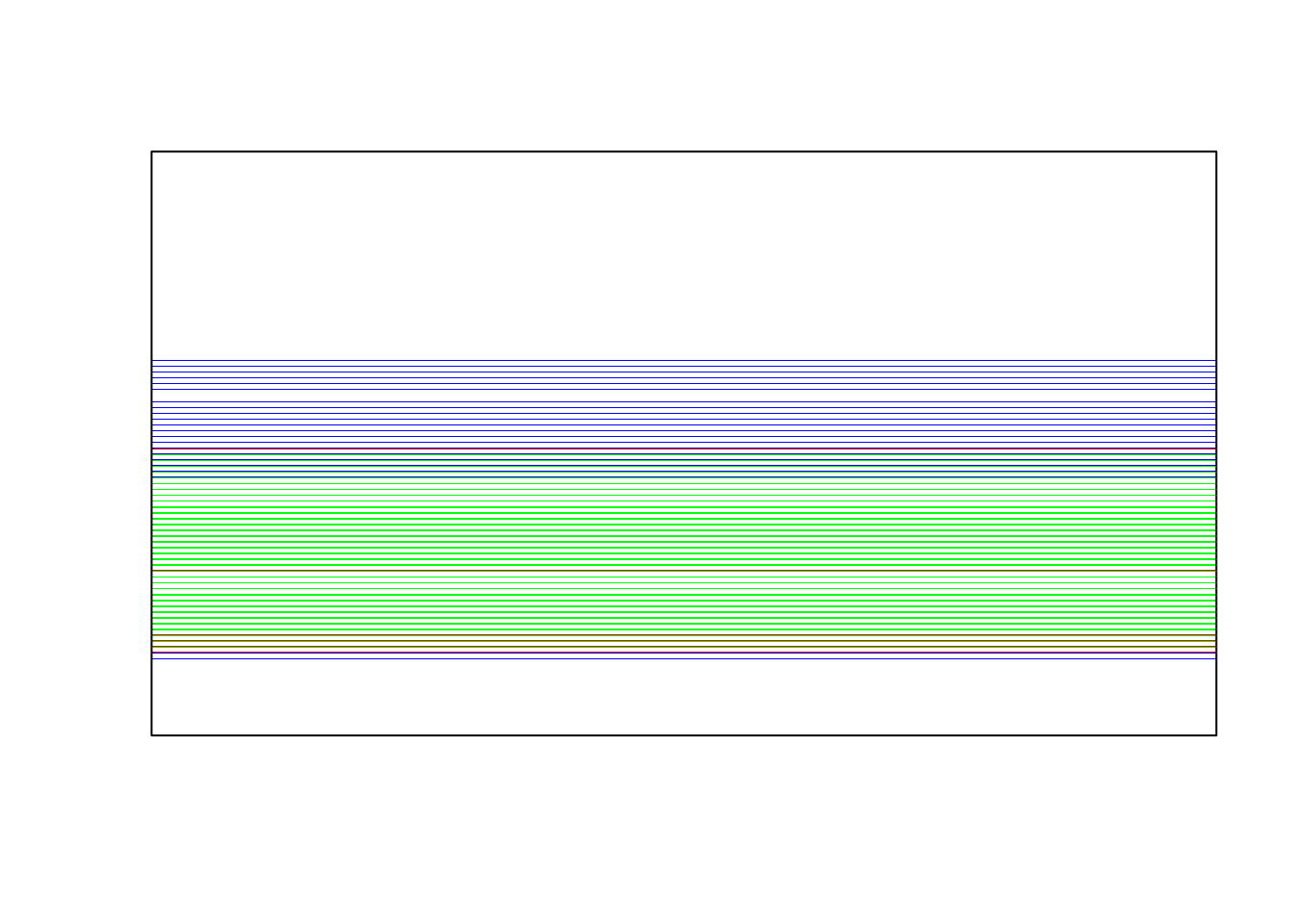For visualization, we can do this all in one operation: cr <- colorRampPalette(c("white", "red", "green", "blue")) plot(2*z1$w+z2$w, col = cr(4)) points(z1$sp, pch = 21, bg = "green", col = 1)
points(z2$sp, pch = 21, bg = "red", col = 1)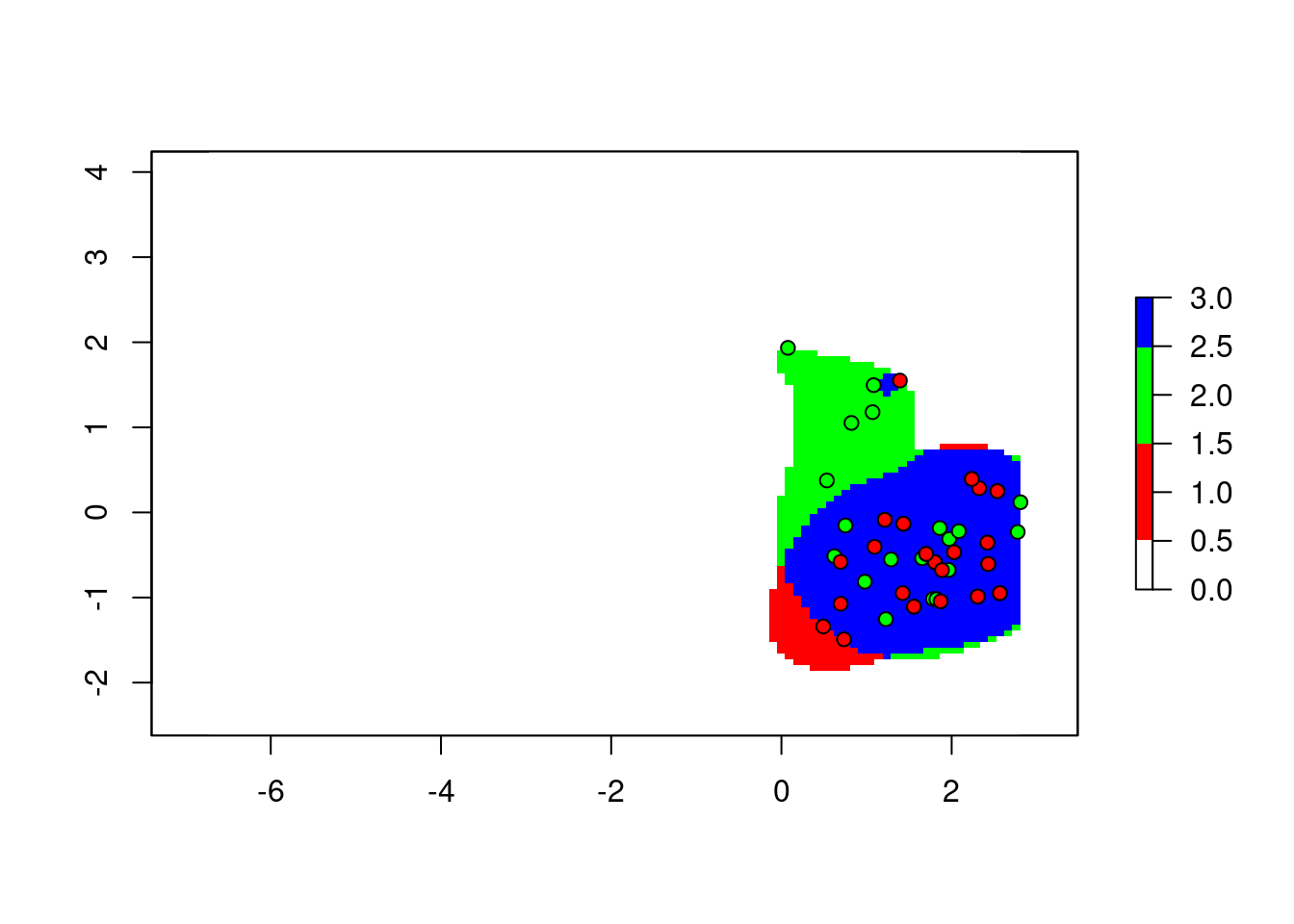To explain this trick: we add the two maps together, with the first map counting twice. The result is, any cell with the value 1 means only the corresponding cell in the second map was occupied. 2 means only the first map is occupied. 3 is both maps occupied. Computation aside, the categorization of pixels is straightforward to interpret. But wait! We can’t just sum up the different categories to get our indices. Each cell is weighted before we compute the indices. Applying the same trick, the calculations proceed as follows: ## Create a raster with the classified pixels: dyn.raster <- 2*z1$w+z2$w ## Extract the three categories: exp.raster <- dyn.raster == 1 unfill.raster <- dyn.raster == 2 stab.raster <- dyn.raster == 3 ## Compute their weights: exp.weighted <- z2$z.uncor * exp.raster
## stability also weighted by species 2:
stab.weighted <- z2$z.uncor * stab.raster ## unfilling weighted by species 1: unfill.weighted <- z1$z.uncor * unfill.raster
## but we need stability weighted by species 1 too:
stab1.weighted <- z1\$z.uncor * stab.raster

## Calculate indices:

expansion.index <- sum(getValues(exp.weighted)) /
sum(getValues(exp.weighted + stab.weighted))

stability.index <- sum(getValues(stab.weighted)) /
sum(getValues(exp.weighted + stab.weighted))
## alternatively, 1 - expansion.index

unfilling.index <- sum(getValues(unfill.weighted)) /
sum(getValues(unfill.weighted + stab1.weighted))

And the values I get from my re-implementation of ecospat match exactly:

My indices: Expansion: 0.060, Stability: 0.940, Unfilling: 0.136

Ecospat indices: Expansion: 0.060, Stability: 0.940, Unfilling: 0.136

flip <- function(x, axis.flip){
x[, axis.flip] <- x[ , axis.flip] * -1
return(x)
}

# References

Broennimann, O., M. C. Fitzpatrick, P. B. Pearman, B. Petitpierre, L. Pellissier, N. G. Yoccoz, W. Thuiller, et al. 2012. Measuring ecological niche overlap from occurrence and spatial environmental data. Global Ecology and Biogeography 21: 481–497.
Calenge, C. 2006. The package ‘adehabitat’ for the R software: A tool for the analysis of space and habitat use by animals. Ecological Modelling 197: 516–519.
Cola, V. D., O. Broennimann, B. Petitpierre, F. T. Breiner, M. D’Amen, C. Randin, R. Engler, et al. 2017. Ecospat: An R package to support spatial analyses and modeling of species niches and distributions. Ecography 40: 774–787.
Phillips, S. J., and J. Elith. 2010. POC plots: Calibrating species distribution models with presence-only data. Ecology 91: 2476–2484.
Worton, B. J. 1989a. Kernel Methods for Estimating the Utilization Distribution in Home-Range Studies. Ecology 70: 164–168.
Worton, B. J. 1989b. Optimal smoothing parameters for multivariate fized and adaptive kernel methods. Journal of Statistical Computation and Simulation 32: 45–57.
Worton, B. J. 1995. Using Monte Carlo Simulation to Evaluate Kernel-Based Home Range Estimators. The Journal of Wildlife Management 59: 794–800.